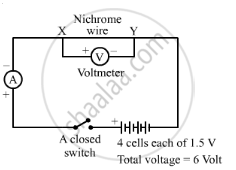Share

# An Electric Circuit Consisting of a 0.5 M Long Nichrome Wire Xy, an Ammeter, a Voltmeter, Four Cells of 1.5 V Each and a Plug Key Was Set Up. (I) Draw a Diagram of this Electric Circuit to Study the Relation Between the Potential Difference Maintained Between the Points 'X' and 'Y' and the Electric Current Flowing Through Xy - CBSE Class 10 - Science

#### Question

An electric circuit consisting of a 0.5 m long nichrome wire XY, an ammeter, a voltmeter, four cells of 1.5 V each and a plug key was set up.

(i) Draw a diagram of this electric circuit to study the relation between the potential difference maintained between the points 'X' and 'Y' and the electric current flowing through XY.

#### SolutionIs there an error in this question or solution?

#### Video TutorialsVIEW ALL 

Solution An Electric Circuit Consisting of a 0.5 M Long Nichrome Wire Xy, an Ammeter, a Voltmeter, Four Cells of 1.5 V Each and a Plug Key Was Set Up. (I) Draw a Diagram of this Electric Circuit to Study the Relation Between the Potential Difference Maintained Between the Points 'X' and 'Y' and the Electric Current Flowing Through Xy Concept: Electric Current and Circuit.
S